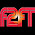## Friday, June 26, 2015

### 33 44 59 115 134 | The Death of Pope John Paul, Reflecting on the Pope's Coming Visit

Pope John Paul was Pope for 33-days, before dying on September 28, 1978.
• 9/28/1978 = 9+2+8+1+9+7+8 = 44 (Kill in Simple English Gematria)
• 9/28/1978 = 9+28+19+78 = 134 (The number of days from Philly Train Crash to Pope's Visit)
• 9/28/78 = 9+28+78 = 115 (Killing in Jewish Gematria)
The name 'Pope John Paul' also ties in with killing.
• Pope = 7+6+7+5 = 25
• John = 1+6+8+5 = 20
• Paul = 7+1+3+3 = 14
• Pope John Paul = 59 (Kill = 59 in Jewish Gematria)
Pope John Paul has some interesting life numbers a well.
• 10/17/1912 = 1+0+1+7+1+9+1+2 = 22
• 10/17/1912 = 10+17+19+12 = 58
• 10/17/12 = 10+17+12 = 39
Pope John Paul II replaced John Paul I on October 16, 1978.
• 10/16/1978 = 1+0+1+6+1+9+7+8 = 33
• 10/16/1978 = 10+16+19+78 = 123
• 10/16/78 = 10+16+78 = 104

1.Hey Zach, Did you know that the first blood shed in the Civil War was in Baltimore April 19, 1861? Freddie Gray died on April 19th and might be connected to that bloody riot. Here are a couple of guys who speak the truth.

1.